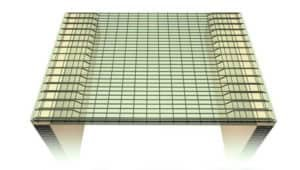# Difference Between One Way Slab And Two Way Slab

## ONE WAY SLAB:

One way slab is a slab which is supported by beams on the two opposite sides to carry the load along one direction. In one way slab, the ratio of longer span (l) to shorter span (b) is equal or greater than 2, i.e Longer span (l)/Shorter span (b) ≥ 2Verandah slab is a type of one way slab, where the slab is spanning in the shorter direction with main reinforcement and the distribution of reinforcement in the transverse direction.

READ -  How To Calculate Cutting Length Of Bent Up Bars In Slab

## TWO WAY SLAB:When a reinforced concrete slab is supported by beams on all the four sides and the loads are carried by the supports along both directions, it is known as two way slab. In two way slab, the ratio of longer span (l) to shorter span (b) is less than 2.

i.e Longer span (l)/Shorter span (b) < 2

This types of slabs are mostly used in the floor of multi-storey buildings.

## Difference Between One Way slabs And Two Way Slabs:

1. In one way slab, the slabs are supported by the beams on the two opposite sides.

In two way slab, the slabs are supported on all the four sides.

2. In one way slab, the loads are carried along one direction.

In two way slab, the loads are carried along both directions.

3. In one way slab, the ratio of Longer span to shorter span is equal or greater than 2. (i.e l/b ≥ 2).

In two way slab, the ratio of l/b is less than 2 (i.e l/b < 2).